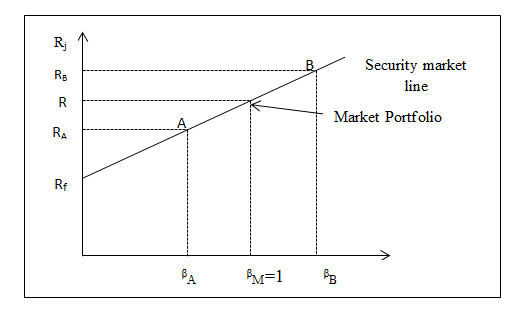## Security Market Line | SML | Capital Assets Pricing Model | Investment Management

### Security Market Line

The security market line is the graphical representation of the capital assets pricing model. The model SML is a graph or line that shows the relationship between the risk-adjusted required rate of return of risky assets and systematic risk measured by beta coefficient. Security Market Line(SML) is the graphical representation of CAPM which shows the relationship between the required return on individual security as a function of systematic, non-diversifiable risk. Security Market Line(SML) measures the risk through beta, which helps to find the security’s risk contribution to the portfolio.

Security Market Line has asset-pricing implication for both portfolios and individual securities. The securities lying above SML is said to be underpriced as it indicates that return from the security is greater than what is required to compensate for systematic risk associated with the security. Similarly all the securities lying below SML is considered to be overpriced as it fails to give sufficient return to compensate against the systematic risk of the security.

On the basis of asset-pricing implication show by SML , investors prefer to buy all underpriced securities and sell overpriced securities. The logic of the SML or CAPM equation is that the required return on any investment is the risk-free return plus a risk adjustment factor. The risk adjustment factor is simply the risk premium required for market return ,multiplied by the riskiness of the individual security.

The slope of Security Market Line reflects the degree of risk aversion on the part of the investors. If the degree of risk aversion is higher for average investors, the slope of security market line is relatively steeper. As a result, risk premium on any security will be larger and consequently the required return will also be higher.

### Illustration,

Suppose the risk-free rate of return is 6%, the expected rate of return on the market is 15 percent, the beta of asset A is 0.5 and the beta of asset B is 1.5. Calculate the required rate of return for each asset.

Solution:

• Rm = 15 %
• Rf = 6%
• βA= 0.5
• βB= 1.5

RA = 6+ (15-6) × 0.5 = 10.5

RB = 6 + (15-6) × 1.5 = 19.5

### Graph of SML

The graphs of the Security Market Line define both efficient and non-efficient portfolios. The Y-axis of the SML represents the level of the required return on individual assets, and the X-axis shows the level of risk represented by beta. The security market line is a positively sloped line displaying the relationship between the required return from security for each given level of non-diversifiable risk(beta).  The slope of the line [E(Rm) – Rf] is the market risk premium. In a well-functioning market/competitive market, a market portfolio is made up of all these assets, so, all the assets must plot on the SML.### Which one of the following is represented by the slope of the security market line?

Options

a. Reward-to-risk ratio

b. Market standard deviation

c. Beta coefficient

d. Risk-free interest rate

### A stock with an actual return that lies above the security market line has:

Options

a. more systematic risk than the overall market.

b. more risk than that warranted by CAPM.

c. a higher return than expected for the level of risk assumed.

d. less systematic risk than the overall market.

e. a return equivalent to the level of risk assumed.

### Which one of the following is the slope of the security market line?

Options

A. Risk-free rate

C. Beta coefficient

D. Risk premium on an individual asset

### The intercept point of the security market line is the rate of return which corresponds to:

Options

A) The risk-free rate of return.

B) The market rate of return.

C) A value of zero.

D) A value of 1.0.

E) The beta of the market.

### Which one of the following is the vertical intercept of the security market line?

Options

a. Market rate of return

b. Individual security rate of return